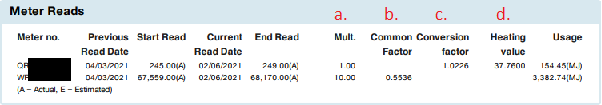### How is my gas usage calculated?

To calculate how much gas has been used over a period of time, subtract the meter reading on the last bill, from the current meter reading.  See the article here for how to read your gas meter.

Gas meters record the volume of gas used in your home. Digital or metric meters record cubic metres and clock face meters record cubic feet

The energy use on your bill has been converted to megajoules (MJ).

If you want to calculate your gas use from reading your meter, you can convert it to megajoules by multiplying the cubic meters or cubic feet of gas used by the multiplier, the common/conversion factor and heating values found on a recent bill.

Below is an example of the meter read sections from our gas bill where all this information can be founda. Multiplier

b. Common Factor

c. Conversion Factor

d. heating value

Use this formula:

• [difference between current and last readings] x [multiplier] x [common factor or conversion factor] x [heating value] = MJ of gas used.

### Costs

Multiplying the amount of gas used in your home by the rates on your gas bill will give you an indication of your energy costs.  Note this formula doesn't include daily supply charges

• [usage] x [rates on your bill ] = estimated cost of gas used between readings.

0 out of 0 found this helpful